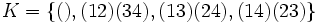# 2-subnormal not implies hypernormalized

This article gives the statement and possibly, proof, of a non-implication relation between two subgroup properties. That is, it states that every subgroup satisfying the first subgroup property (i.e., 2-subnormal subgroup) need not satisfy the second subgroup property (i.e., hypernormalized subgroup)
View a complete list of subgroup property non-implications | View a complete list of subgroup property implications
EXPLORE EXAMPLES YOURSELF: View examples of subgroups satisfying property 2-subnormal subgroup but not hypernormalized subgroup|View examples of subgroups satisfying property 2-subnormal subgroup and hypernormalized subgroup

## Statement

### Verbal statement

A 2-subnormal subgroup of a group need not be hypernormalized.

## Proof

### An example in the symmetric group on four letters

Let$G$ be the symmetric group on four letters$\{ 1,2,3,4\}$ and$H$ be the two-element subgroup generated by$(13)(24)$.

Then,$H$ is normal in the subgroup$K = \{ (), (12)(34), (13)(24), (14)(23)\}$, which is normal in$G$. So$H$ is 2-subnormal in$G$.

On the other hand, the normalizer$N_G(H)$ is a dihedral subgroup of order eight, which is a self-normalizing subgroup.